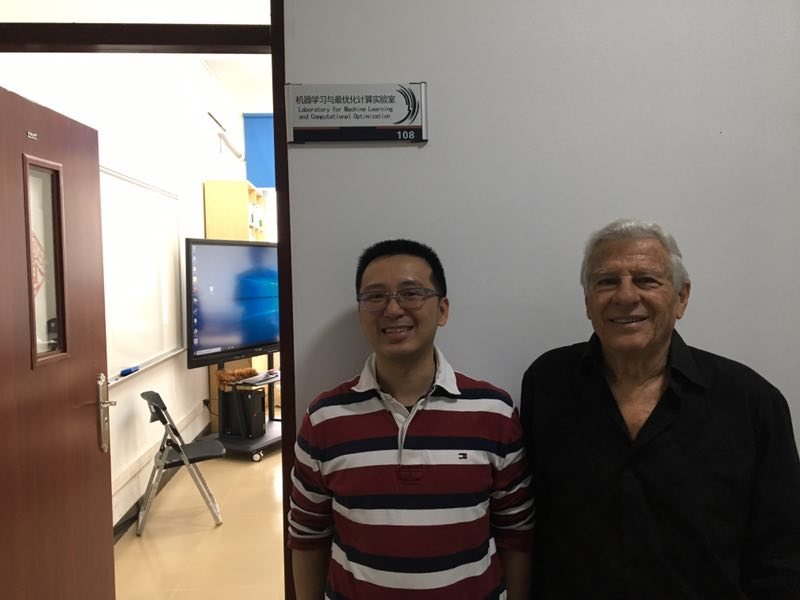# Dany Leviatan教授受邀进行讲座

Dany Leviatan教授是国际著名逼近论专家，在国际发表上百篇学术文章，担任多本著名数学杂志的编辑，曾任以色列特拉维夫大学数学系的系主任和特拉维夫大学的校长（rector）。

Given a monotone or convex function f, we obtain pointwise estimates for its monotone,respectively, convex approximation by piecewise polynomials involving the second order modulus of smoothness of f^r. These estimates are interpolatory estimates, namely, the piecewise polynomials interpolate the function at the end-points of the interval. However,they are valid only for n > N(f,r). We also show thatsuch estimates are in general invalid with N independent of f. Note that the above estimate in the case r = 0 is achieved by piecewise linears interpolating f at the Chebyshev nodes. This was proved in the early 1980's by DeVore and Yu (for monotone approximation) and by Leviatan (for convex approximation).